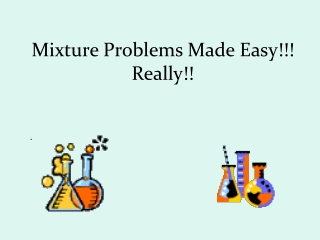Download PresentationMixture Problems Made Easy!!! Really!!

# Mixture Problems Made Easy!!! Really!!

Download Presentation## Mixture Problems Made Easy!!! Really!!

- - - - - - - - - - - - - - - - - - - - - - - - - - - E N D - - - - - - - - - - - - - - - - - - - - - - - - - - -
##### Presentation Transcript

1. Mixture Problems Made Easy!!! Really!! .

2. How many liters of a solution that is 20% alcohol should be combined with 10 liters of a solution that is 50% alcohol to create a solution that is 30% alcohol? What are the important parts here? Who would like to come and high light the important components to this problem while the rest of you do it in your notes?

3. This is the magic grid that will lead you to the equation!!. Once you have filled in the grid you are looking at the equation in the last column.

4. Fill the third column with the total amounts in each container. We will use x for the amount in the second container. 10 .50 .20 x .30 x + 10

5. Fill the last column with the products of the first and second columns. Focus on the row for the original. If you multiply the percent strength by the total amount, you will have the amount of alcohol in the last column. .50 5 10 .20 x .20x x + 10 .30(x+10) .30

6. The amount of alcohol in the original plus the amount of alcohol in the added equals the amount of alcohol in the final mixture. The equation is in the final column. .50 10 5 .20 x .20x .30 x + 10 .30(x+10)

7. So here is the final equation to solve which should be quite easy for all of us to solve! 5 + .20x = .30(x + 10). 10 (5 + .20x) = 10 (.30x + 3) 50 + 2x = 3x + 30 -2x -2x 50 = x + 30 -30 -30 20 L = x It takes 20 liters of a 20% solution to dilute 10 liters of a 50% solution to a final solution of 30%.

8. Example 2. How many liters of a solution that is 30% antifreeze should be added to how many liters of a solution that is pure antifreeze to create 5 liters of a solution that is 50% antifreeze? Round your answer to the nearest tenth of a liter. Notice a couple of differences between this Example 1 and Example 2. In the first place, what do you think we are going to use as the percentage strength for pure antifreeze? Think before you click.

9. If you guessed 100%, pat yourself on the back. Secondly, if we let x be the number of liters of the 30% solution, what do you think we should use as the number of liters at 100%? Think before you click. Hint: the total number of liters has to be 5. If you guessed 5 – x, congratulations!

10. Try to fill in what you know. We are going to let x be the number of liters of the 30% solution. We also know that the mixture will total 5 liters .30 X 5

11. . So let us see what we have here now? What are we going to use as an algebraic name for the number of liters of pure antifreeze? Since the total number of liters has to be 5, subtract the number of liters at 30% and get: .30 x 1.00 5 - x .50 5

12. Multiply percent strength by the amount of solution and get the amount of the target ingredient (antifreeze). The third column is your equation. Solve it before you click. .30 x .30x 1.00 5 - x 1(5 – x) .50(5) .50 5

13. From the third column, the equation is: .30x + 1.00(5 – x) = .5(5) Let us continue multiplying through by 10 to get rid of those decimals (10) ( .30x + 1.00 (5 – x )) = (.5 (5) ) (10) 3x + 10(5 - x) = 5(5) 3x + 50 -10x = 25 -7x + 50 = 25 -50 -50 -7x = -25 X = 3.6 5 – x = 1.4 liters

14. We will introduce our final example with a little common sense. It is also pretty interesting. How much water would you have to add to dilute 4 liters of a 70% acid solution down to a 35% acid solution? Think before you click. Congratulate yourself if you guessed 4 liters. Another 4 liters would dilute the original 4 liters to half of its original strength. See if you can work this problem on your own before you click to the solution. Guess first, then use the grid to solve algebraically. Example 3. How much water would you have to add to dilute 4 liters of a 75% acid solution down to a 25% acid solution?

15. The target ingredient will be acid. The second container will be the water. Did you use 0% for the strength of the added column?

16. .75(4) = .25(4 + x) (100) .75(4) = .25(4 + x) (100) 75 (4) = 25 (4 + x) 300 = 100 + 25x 200 = 25x 8 = x It takes 8 liters of water to dilute 4 liters of 75% acid down to 25% strength. Now you may practice with your home work problems. Make sure to refer to your notes if something is not clear. Good luck and I know you will do fine.